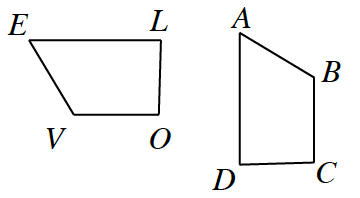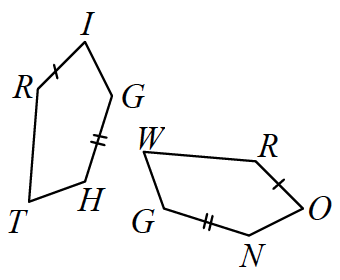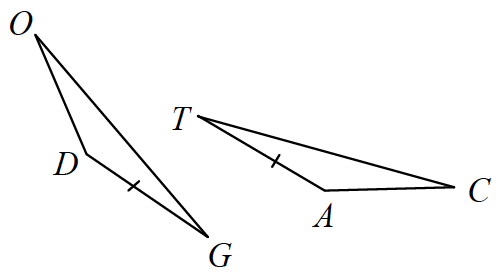### Home > GC > Chapter 3 > Lesson 3.2.1 > Problem3-52

3-52.

Assume that each pair of figures below is similar. Write a similarity statement to illustrate which parts of each shape correspond. Remember: letter order is important!

1. $ABCD\sim$Which parts of these similar shapes relate to each other?

$ABCD\sim EVOL$

1. $RIGHT\sim$Use the same method as you did for part (a).

1. $\Delta$_____ $\sim$ $\Delta$_____Name the first triangle in any order. Then determine the order in which the second triangle should be named.